Disclosure: This post may contain affiliate links, meaning when you click the links and make a purchase, we receive a commission.

# How to use Goal Seek in Excel 2016 [Solve 3 Problems]

If you know the result of a formula, but you are not sure which input value the formula needs to get that result, you can use the Goal Seek Command.

Suppose that you want to pay off your mortgage in 15 years, the annual interest rate is 6 percent and you can afford to pay only \$2,000 each month, then how much you can borrow?

Problems similar to this in real life can be solved using Goal Seek feature as long as the real-world problems can be modeled into one variable equation.

In above example, we can model it into below equation:

Monthly Payment = PMT(Interest Rate/12, Mortgage Term Length in Months, Mortgage Amount)

By substituting with real numbers, we will get an equation like following:

\$2,000 = PMT(6%/12,180,Mortgage Amount)

Now we have a formula, the result of a formula and an unknown input value. Looking back at the first sentence of this article, you will see this is exactly the problem that we can use Goal Seek to solve.

Essentially, Goal Seek embeds a powerful equation solver in a worksheet. This equation solver requires us to provide three pieces of information: a formula, result of that formula and a variable which can contain unknown input value. Here I have to remind you that we need to put required information into cells as Microsoft Excel can only manipulate cells.

Now let’s move forward and look at how to use Goal Seek command to solve real-world problems.

Read more: Goal Seek in Excel 2013 – How to Use Guide

## Case 1: Let’s solve a mortgage problem

Suppose that you need to borrow \$300,000 from a bank for 30 years to buy a house. If your maximum payments are limited to \$1,500 per month, how high an annual interest rate can you tolerate?

We can model this situation using this formula – \$1,500 = PMT(Interest Rate/12,360,\$300,000).

In order to show you how to put those three essential pieces of information into Excel, I established a table as shown Figure 1.1. Cell C5 contains formula “=PMT(C4/12,C3,C2)” and Cell C4 represents for a variable which contains the unknown input value. Cell C4 was left blank because we don’t know the interest rate. The formula still returned a value, though cell C4 does not have value. Why? Because PMT function assumes a 0% annual interest rate in this case.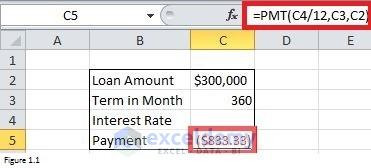Figure 1.1

You must have noticed that we already have Cell C5 and Cell C4 to contain a formula and changing variable respectively. Where can we put the result of the formula, \$1,500? We need to put in Goal Seek dialog box. To open this dialog box, we need to click Data tab at first, then click on What-If Analysis and finally choose Goal Seek in the drop-down (Please see Figure 1.2 for details).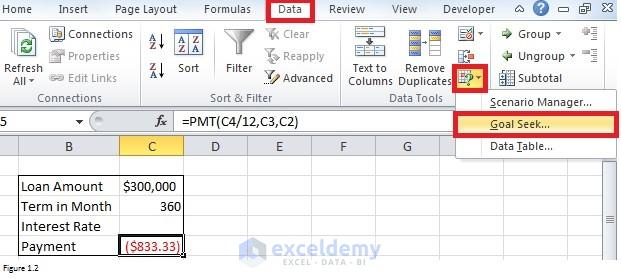Figure 1.2

After clicking Goal Seek, the Goal Seek dialog box showed in Figure 1.3 will be prompted. In the Set cell box, enter C5. And in the To value box, type your payment amount, -1500. Please note that the number must be negative because it’s a payment. Finally, in the By changing cell box, enter C4 or \$C\$4, the reference to the cell that contains the value that you want to adjust. Please remember that the cell you enter here must be referenced by the formula that you specify in the Set cell box.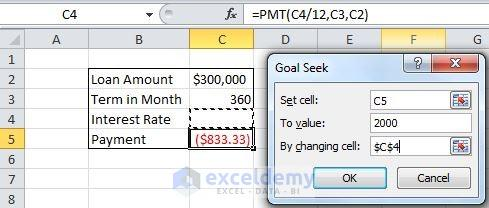Figure 1.3

After clicking OK, the Goal Seek dialog box will change into status like below telling that Excel found a solution for us. And you can see that cell C4 is no longer blank. Excel put the solution, 4.39%, in cell C4. It means that you have to pay more than \$1,500 each month if the interest rate is higher than 4.39%. Therefore the highest interest rate that you can tolerate is \$4.39%.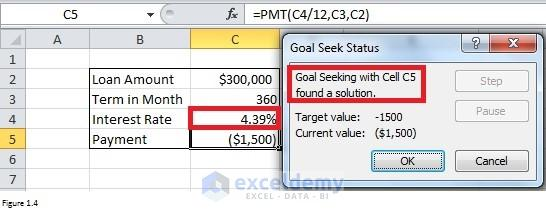Figure 1.4

## Case 2: Get back to school (solve an algebra problem)

Most story problems in high school algebra require you to choose a variable (which is usually called x) to solve a particular equation. This is another situation in which we use Excel Goal Seek feature? Here is a typical high school story problem. Suppose that you are buying 40 pounds of candy. Some of the candy sells for \$10 per pound, and some sell for \$6 per pound. How much candy at each price should you buy to result in an average cost of \$7 per pound?

Read More: How to use Goal Seek to find an input value & produce a desired result

Let’s connect this situation with an equation first. In order to describe in an easier way, I will give two kinds of candies a name respectively. The candy which sells for \$10 per pound will be called Candy A and another one which sells for \$6 will be called Candy B. Variable x represents how many pounds of Candy A that we will buy. (40 – x) represents how many pounds of Candy B that we will buy. Formula x*10 + (40 – x)*6 can tell how much money we have to pay for these candies. Diving above formula by 40, we can get the average cost. In summary, the equation will be:

(x*10 + (40 – x)*6)/40 = 7

Following Figure 2.1 shows how to put required information in Excel and build the formula. Please note that the formula “=(C2*10+(40-C2)*6)/40” returns 6 as it assumes that the value of cell C2 is 0.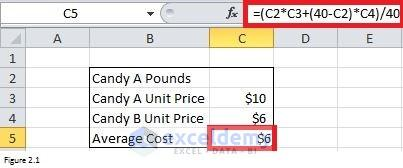Figure 2.1

But entering C5 in Set cell field, 7 in To Value box and C2 in By changing cell, we can see that a value 10 was put in Cell C2 and the average cost will change into 7 which is what we are seeking.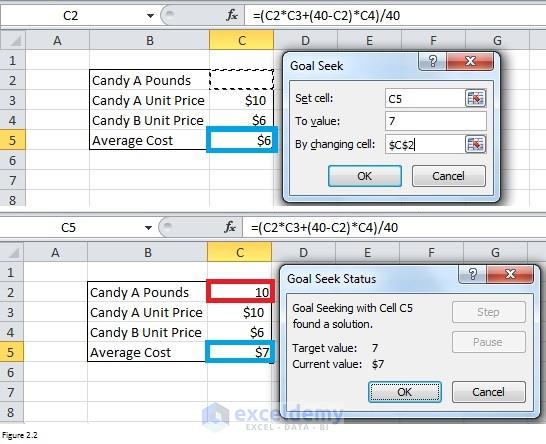Figure 2.2

## Case 3: Conference Registration Problem

You are managing a conference at your college. Your fixed costs are \$15,000. You must pay the 10 speakers \$700 each and the college union \$300 per conference participant for food and lodging costs. You are charging each conference participant who is not also a speaker \$900, which includes the conference fee and food and lodging costs. How many paid regis­trants need to attend for you to break even?

Read More: Financial Planning with Excel Solver [2 Case Studies]

To break even, your gross income minus your cost should be equal to 0. Therefore, we can write a formula in this way:

Number of non-speaker * \$900 – (\$15,000 + \$300 + 10 * \$700) = 0

Figure 3.1 shows how to put information into Excel and build a formula. The current net revenue is -\$22,300 because we do not have any gross income.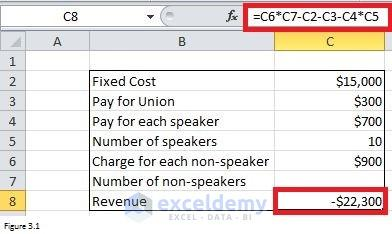Figure 3.1

By putting C8 in Set cell box, 0 in To value box and C7 in By changing cell box, the Goal Seek command returns a number which tells that you need 25 non-speaker participants to break even.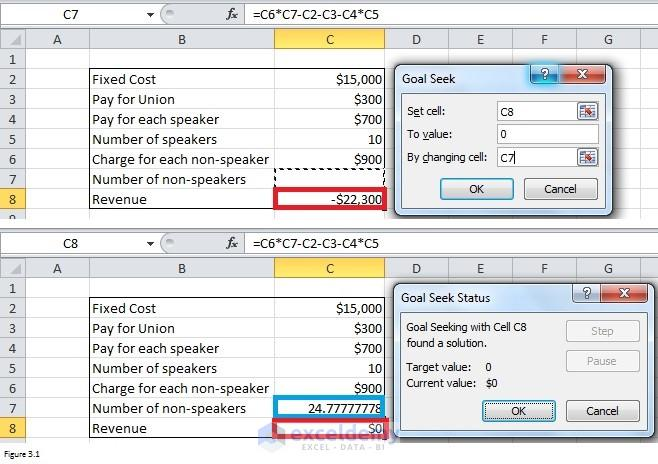Figure 3.2